Case Based Questions (MCQ)

Chapter 13 Class 10 Statistics
Serial order wise

Question

The maximum bowling speeds, in km per hour, of 33 players at a cricket coaching center are given as follows.

 Speed (in Km/h) 85 − 100 100 − 115 115 − 130 130 − 145 No. of Players 11 9 8 5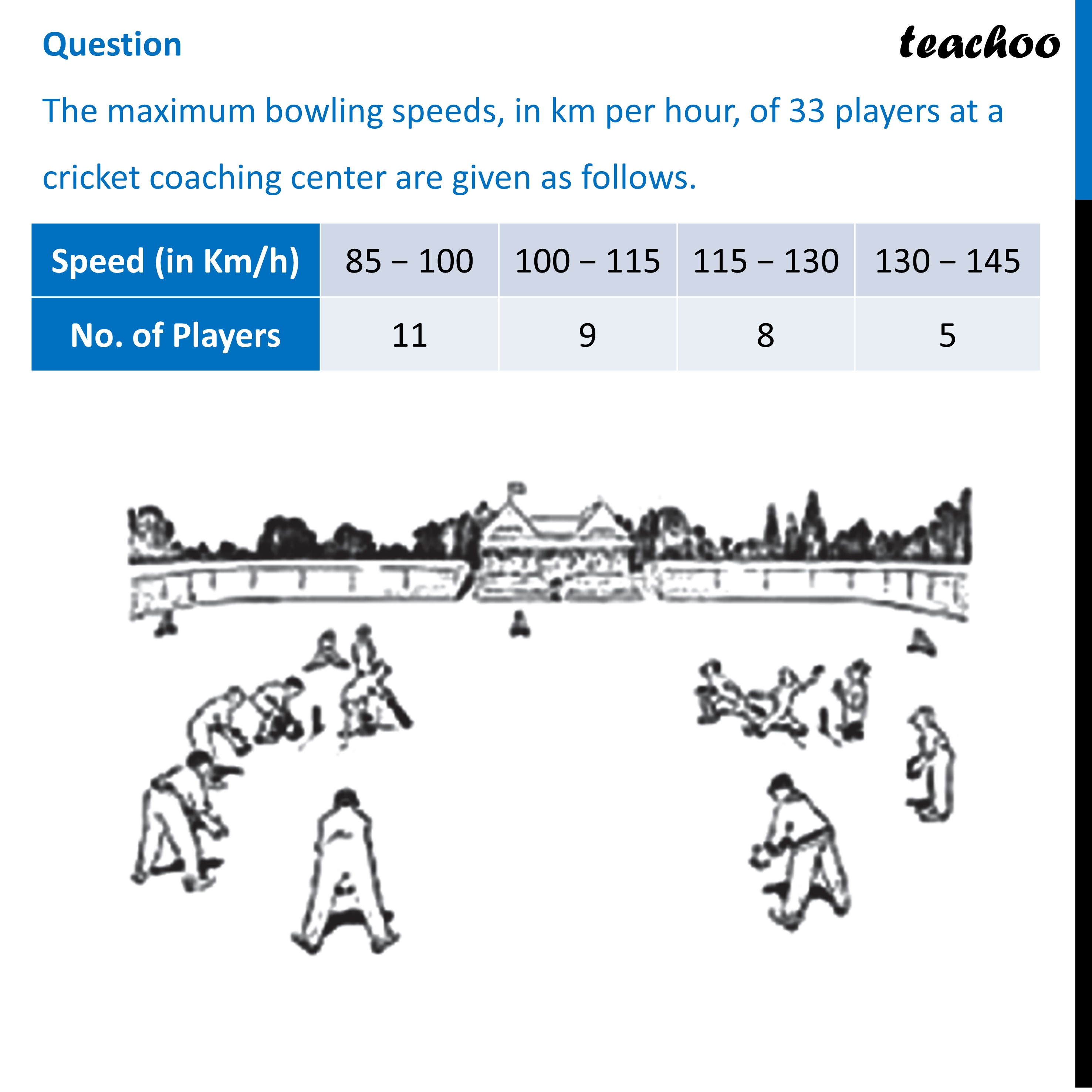## (d) 130-145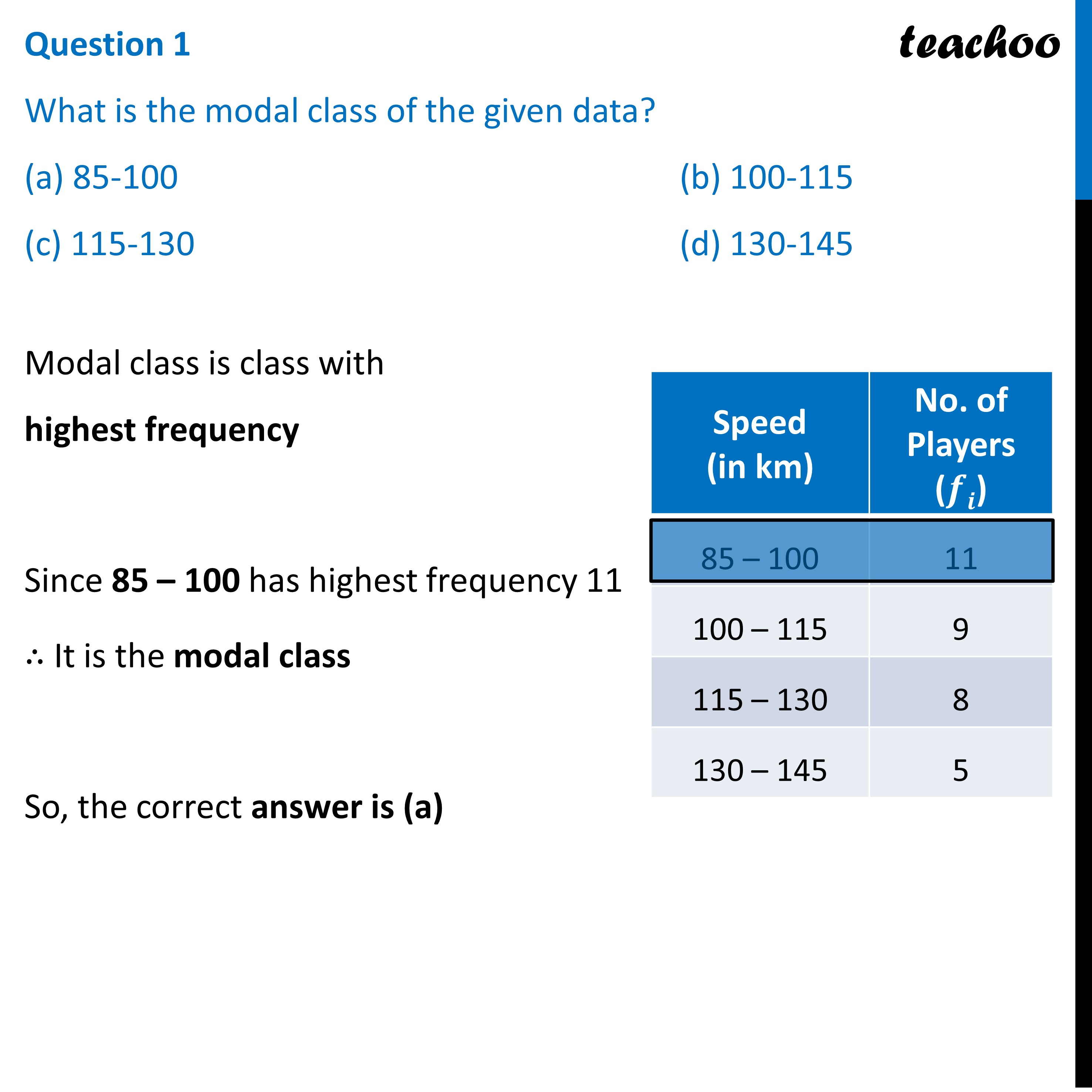## (d) 20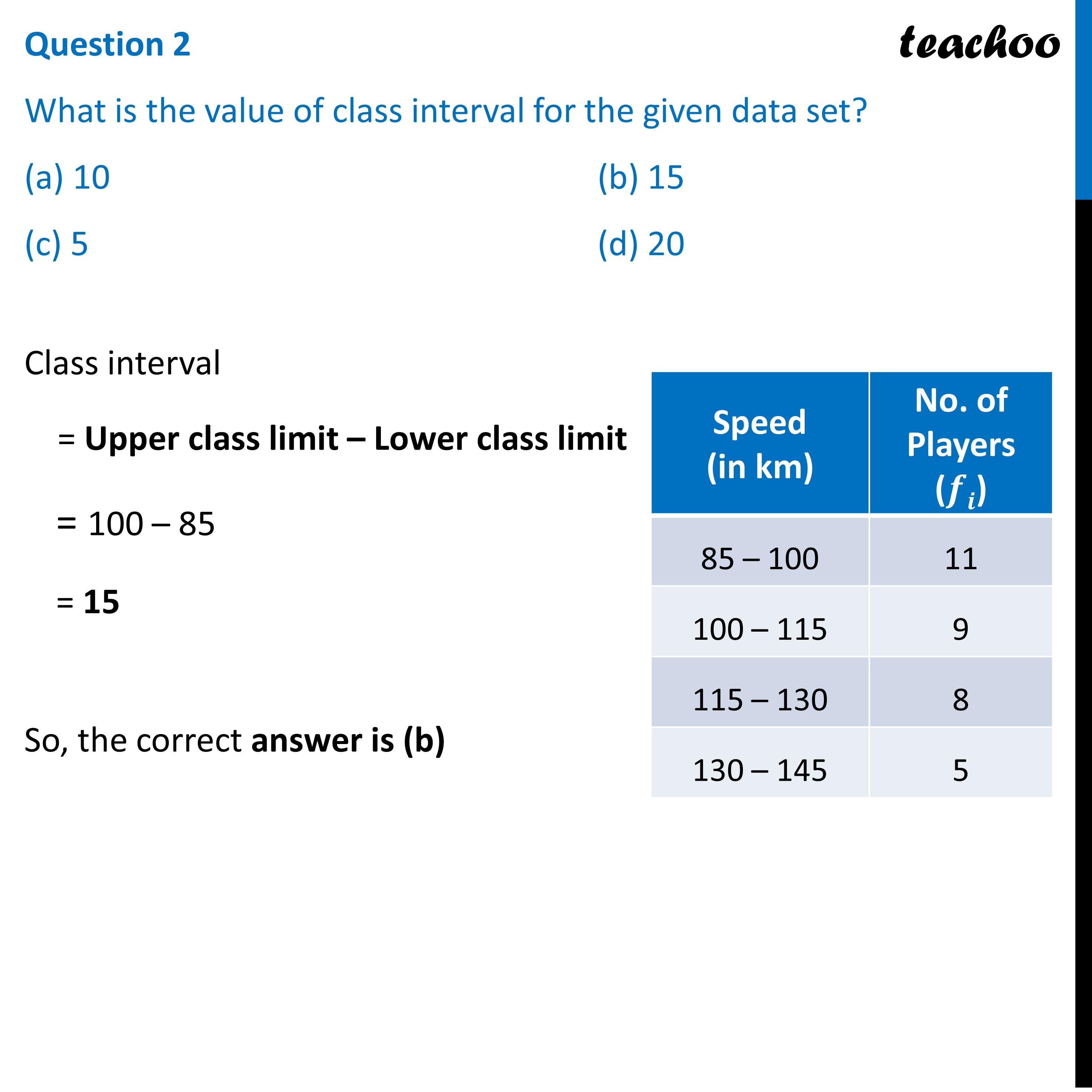## (c) 115-130   (d) 130-145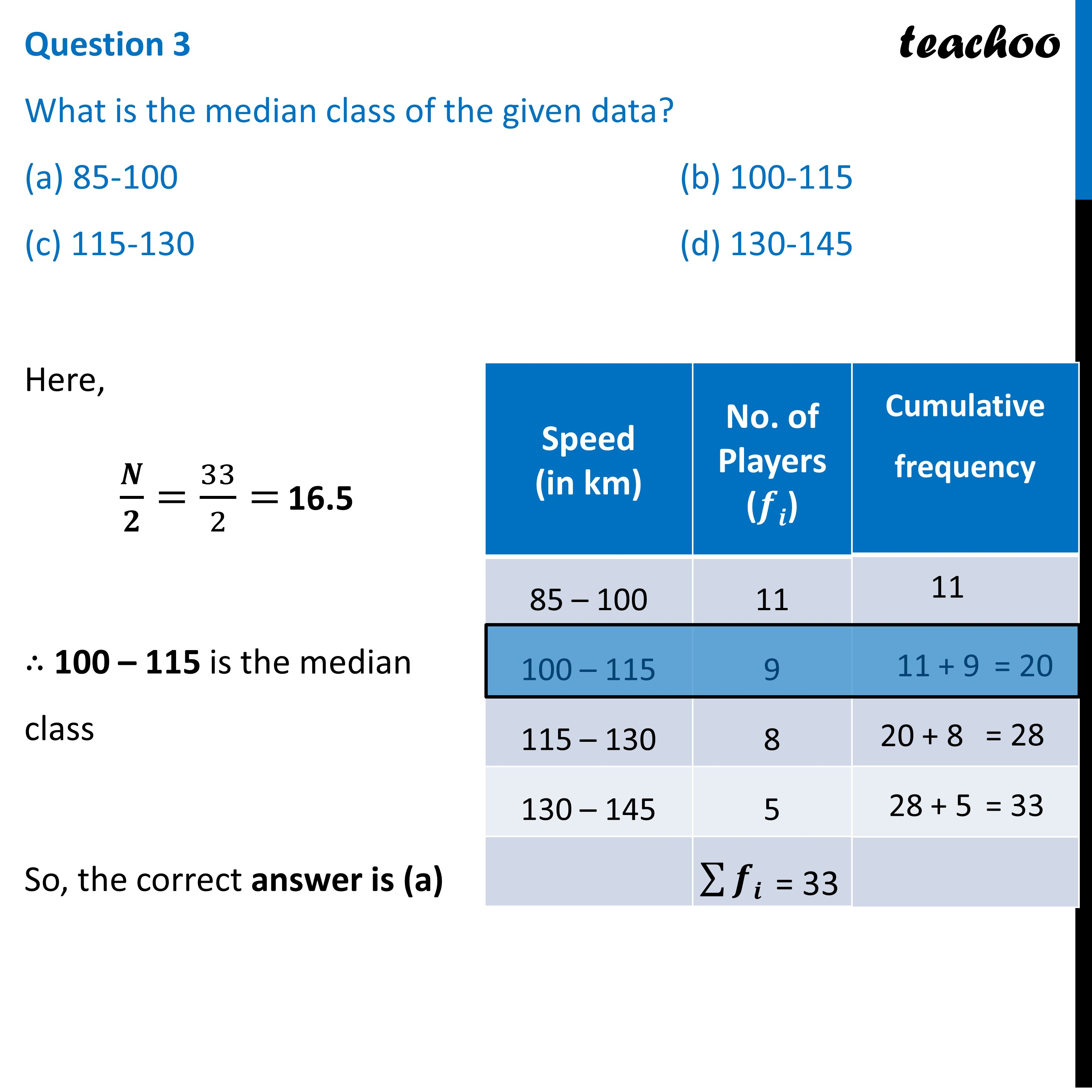## (d) 109.19 km/hr (Approx)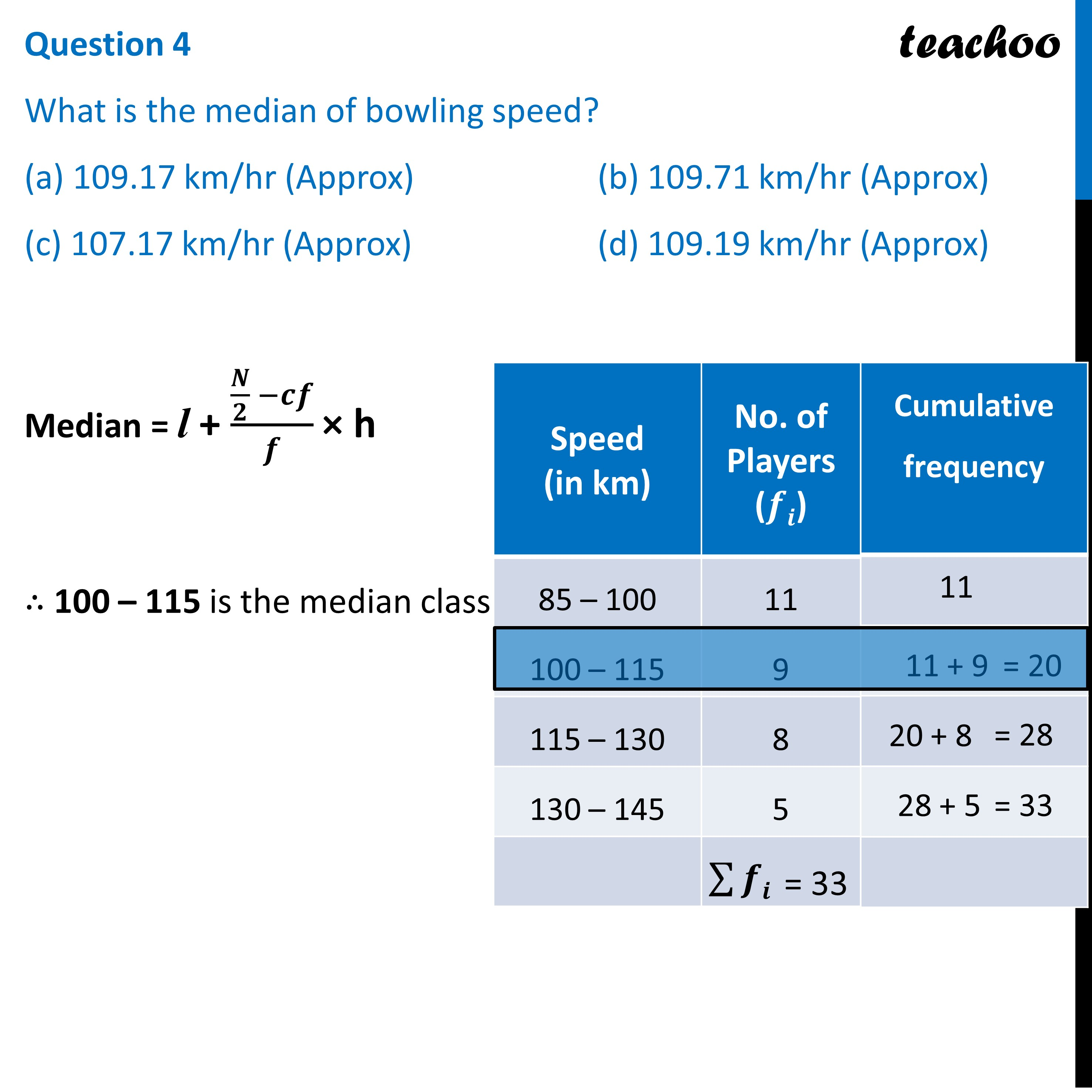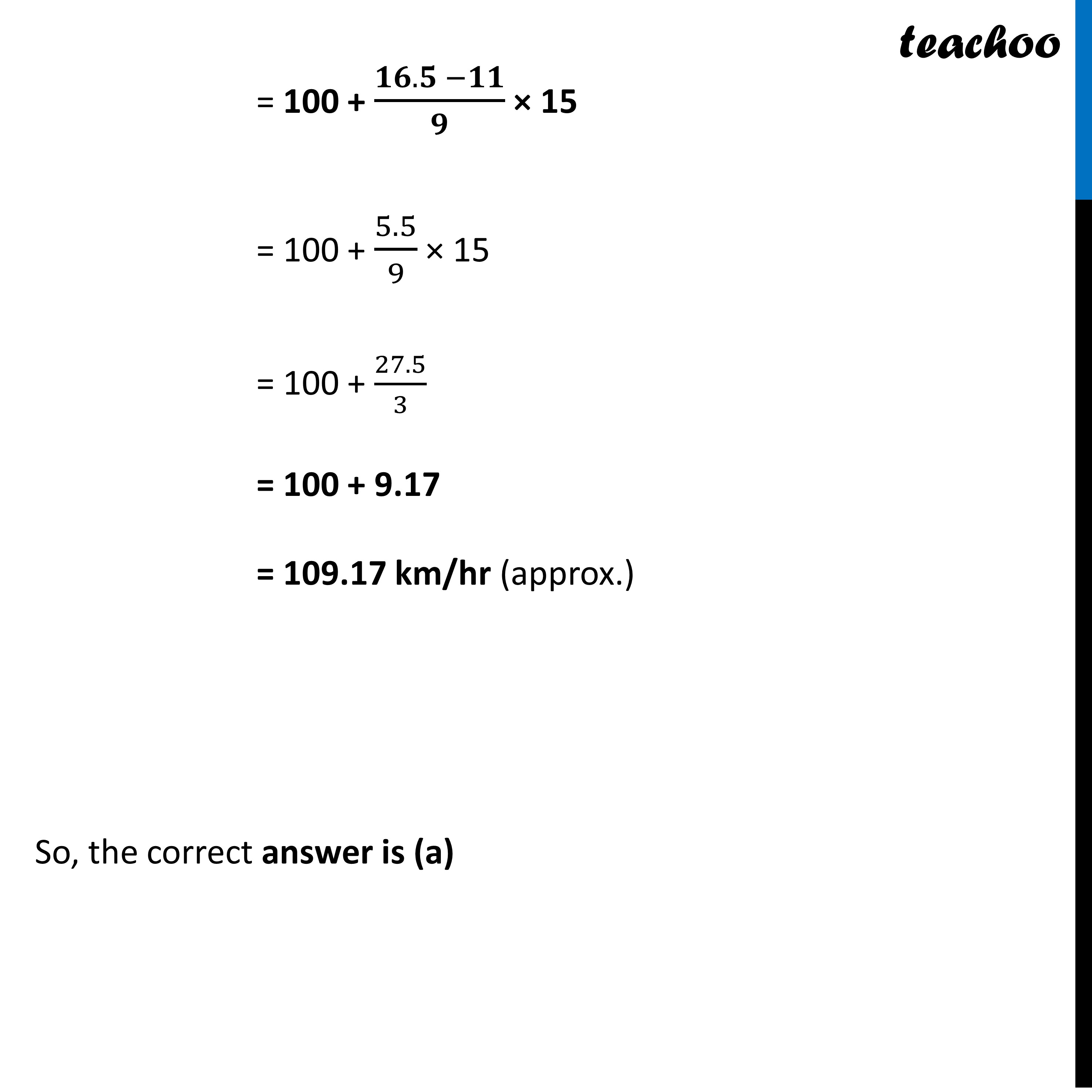## (d) 400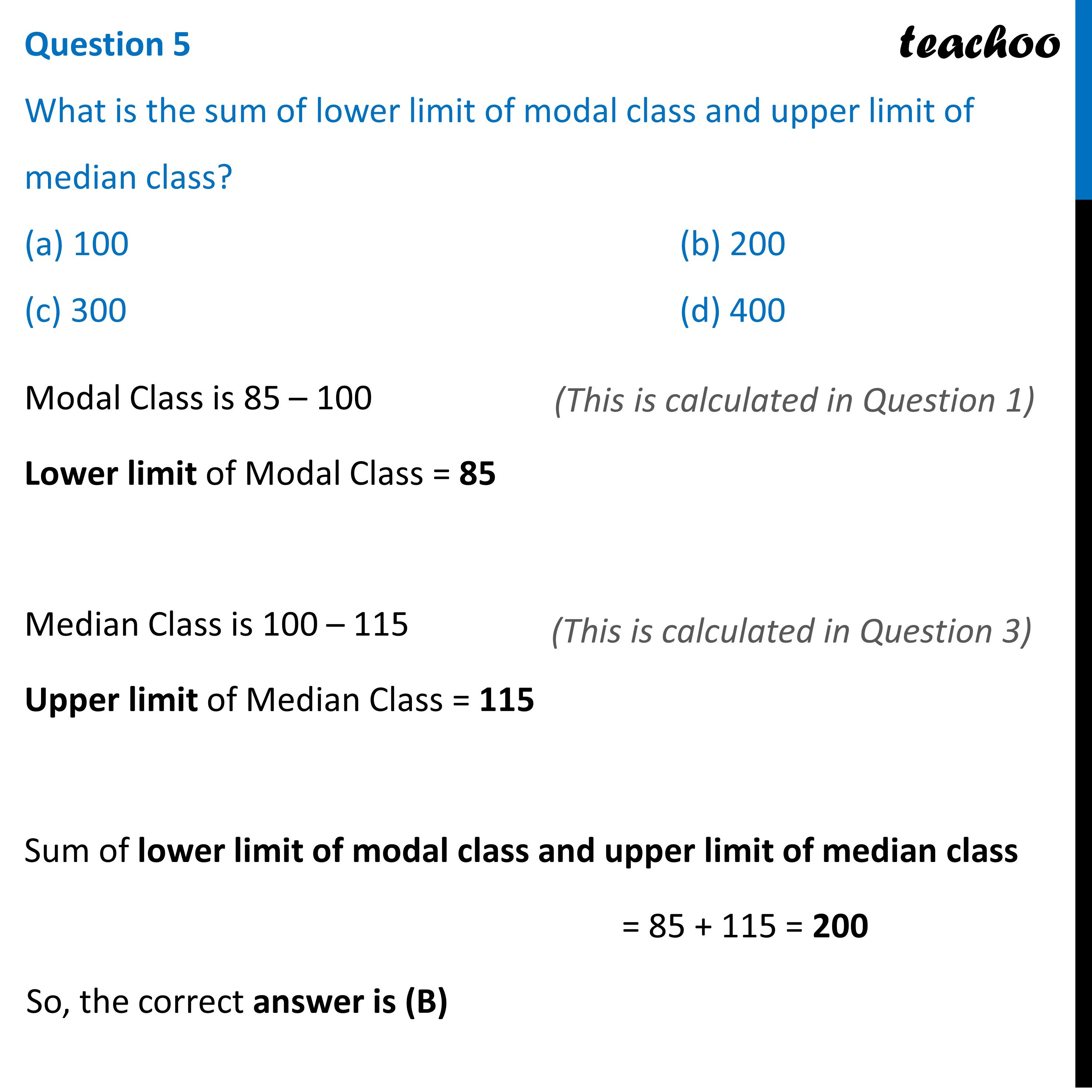Learn in your speed, with individual attention - Teachoo Maths 1-on-1 Class

### Transcript

Question The maximum bowling speeds, in km per hour, of 33 players at a cricket coaching center are given as follows. Question 1 What is the modal class of the given data? (a) 85-100 (b) 100-115 (c) 115-130 (d) 130-145 Modal class is class with highest frequency Since 85 – 100 has highest frequency 11 ∴ It is the modal class So, the correct answer is (a) Question 2 What is the value of class interval for the given data set? (a) 10 (b) 15 (c) 5 (d) 20 Class interval = Upper class limit – Lower class limit = 100 – 85 = 15 So, the correct answer is (b) Question 3 What is the median class of the given data? (a) 85-100 (b) 100-115 (c) 115-130 (d) 130-145 Here, 𝑵/𝟐=33/2= 16.5 ∴ 100 – 115 is the median class So, the correct answer is (a) Question 4 What is the median of bowling speed? (a) 109.17 km/hr (Approx) (b) 109.71 km/hr (Approx) (c) 107.17 km/hr (Approx) (d) 109.19 km/hr (Approx)Median = l + (𝑵/𝟐 −𝒄𝒇)/𝒇 × h ∴ 100 – 115 is the median class And, l = lower limit of median class = 100 h = class-interval = 15 cf = cumulative frequency of the class before median class = 11 f = frequency of the median class = 9 Putting values in formula Median = l + (𝑁/2 −𝑐𝑓)/𝑓 × h = 100 + (𝟏𝟔.𝟓 −𝟏𝟏)/𝟗 × 15 = 100 + 5.5/9 × 15 = 100 + 27.5/3 = 100 + 9.17 = 109.17 km/hr (approx.) So, the correct answer is (a) Question 5 What is the sum of lower limit of modal class and upper limit of median class? (a) 100 (b) 200 (c) 300 (d) 400 Modal Class is 85 – 100 Lower limit of Modal Class = 85 Median Class is 100 – 115 Upper limit of Median Class = 115 Sum of lower limit of modal class and upper limit of median class = 85 + 115 = 200 So, the correct answer is (B)